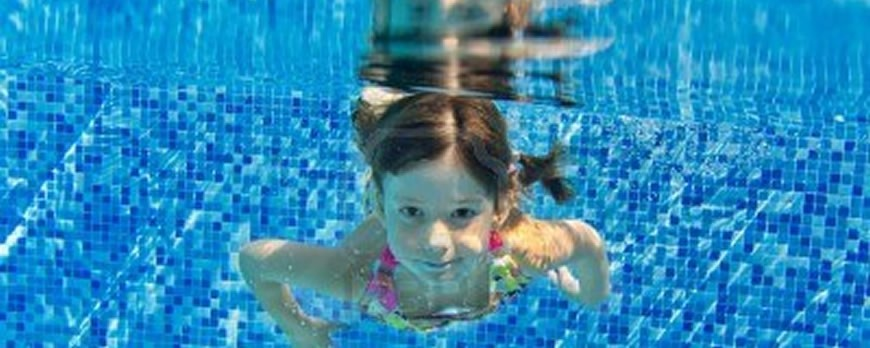HOW TO MEASURE A REPLACEMENT LINER

How to measure a Basic Shape (Rectangle, Grecian, Oval, True L, or Lazy L) liner

It is easier to measure a pool when it is full of water. It is best if the water is clear so you can see the break points. If the water is green and you can’t see the bottom, the following instructions still apply, but you will have to feel for the breaks with the vacuum pole. If you can’t feel where the breaks are, drain the pool. These instructions apply even is the pool is empty.

2. You will need a 50 ft tape measure, a 16 or 25 ft tape measure, the pool vacuum pole, a pencil or pen, some electrical or duct tape, and the liner order form.
3. Stretch the 50 ft tape (on the deck) along the length of the pool with the (0) end of the tape aligned with the shallow end wall. If your pool has 2 or 4 ft radius corners, use the vacuum pole as a straight edge to align the end of the tape with the shallow end wall. You can also lie down on the deck, close one eye, and align the end of the tape as if you were shooting pool.
4. Do the same thing at the deep end wall to determine the total length of the pool.
5. Fill in this measurement (B) on the order form. Leave the tape measure in place.
6. Walk to the point where the shallow end meets the top of the slope. Look straight down at the break and record this measurement (E) on the order form. Place a piece of tape or make a mark on the deck at this point.
7. Walk down the length of the pool to the bottom of the slope. Stand the vacuum pole in the deep end at the point where the slope meets the hopper bottom (flat part of the deep end) and lean it against the coping at the side of the pool. This will help you determine the (N) dimension. Measure from the tape or mark at the shallow end break (E) to the vacuum pole. This is your (N) dimension. Place tape or mark here also.
8. Now move the vacuum pole to where the hopper bottom meets the back wall sloping down to the hopper bottom and lean it against the coping as you did in step 6. This is your (M) dimension. Place tape or mark here also.
9. Using the 16 ft tape, measure from here to the deep end wall of the pool (on the 50 ft tape). This is your (L) dimension.
10. If you add up the length of the shallow end, the length of the slope, the length of the hopper bottom, and the length of the back wall, they should equal the total length of the pool.

(E) + (N) + (M) + (L) = (B)

11. Repeat the same process across the width of the pool at the deep end to determine the left side wall (G), hopper width (H), and right side wall (I) dimensions.

(G) + (H) + (I) = (A)

12. Using the 16 ft tape, measure the depth of the shallow end up to where the liner snaps in the coping (not to the top of the deck). This is your (J) dimension.
13. Using the vacuum pole measure the depth of the water in the deep end. This is easy if you can walk out on the diving board. Make a mark on the pole at water’s depth, then measure with the 16 ft tape. Now, at the edge of the pool measure from the top of the water to where the liner snaps in the coping. Add these two dimensions together to determine the depth of the deep end (K).
14. Most rectangle pools have 6 inch, 2 ft, or 4 ft Radius corners.
• A 6 inch radius is the same size as a 12 inch circle. A 33 1/3 rpm record album is a 6 inch radius. The distance around the curve of a 6 inch radius corner is about 9 ½ inch.
• A 2 ft radius is the same size as a 4 ft circle. The distance around the curve of a 2 ft radius corner is about 37 ½ inch.
• A 4 ft radius is the same size as an 8 ft circle. The distance around a 4 ft radius corner is about 6 ft 3 inch.

After you have completed filling out the liner order form, transfer this information to the uploadable form on the website and give us your contact information. We will call you and go over the information to verify accuracy. We will also help you determine which gaskets, faceplates, screws, etc you may need to complete the job. We are always available by phone 8 to 5 Mon thru Fri. if you have questions during the installation.

General A-B Point to Point Set Up

The first thing to be done is establish 2 points, an A point and a B point approximately 5' from the edge of the pool. The points should be roughly 2/3 of the length of the pool apart. If, for example, your pool is 40' long your distance between A and B should be about 26' apart. The points should be on a flat surface.

Your A and B points if extended with an imaginary line from both ends should not cross the pool at any point. If this line does cross the pool you will have to adjust your points.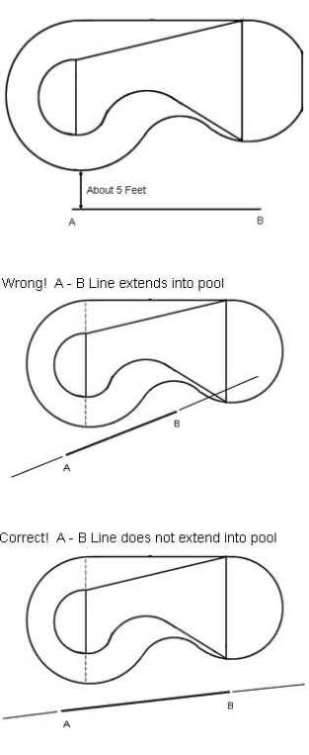Return

Marking A-B Points

To establish your perimeter points mark the points approximately 2' apart along the coping. The distance between points can vary. If you are marking points around a sharp radius you want to mark the points closer. If you are marking points along a straight section you can mark them further apart.

It is important that you mark transition points where a radius starts or ends and also where two straight sections come together at an angle. If your pool has a step or a step cut you need to mark where the step starts and ends.

To help establish the bottom dimensions you need to extend a line across the shallow end break and also across the hopper break line. Mark these points where they meet with the perimeter. One of the shallow end points should be used as point number 1. The other 3 points should be labeled X, Y, and Z. The remainder of your points should be numbered sequentially starting at point number 1.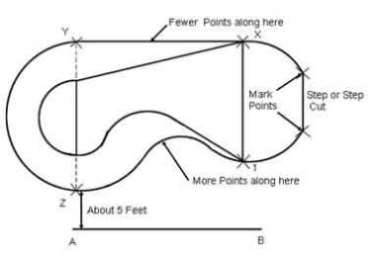Return

Measuring A-B Points

When measuring your points, it is important to make sure that you measure in a straight line, do not bend the tape measure around objects. Make sure that the tape measure is pulled tight. You can round your measurements to the nearest inch.

Measure from point A to all points on the perimeter and then from point B to all points on the perimeter. You will also need to find the overall length and width of the pool. To do this you will need to pick a point in the shallow end and a point in the deep end. Select points that will best approximate the overall length of the pool. Note which points were used and what the measurement was. Use the same method to find the overall width of the shallow end and deep end.

It is best to leave your points including the A and B points until the liner is installed. That way if there are questions or problems we can refer back to the original points.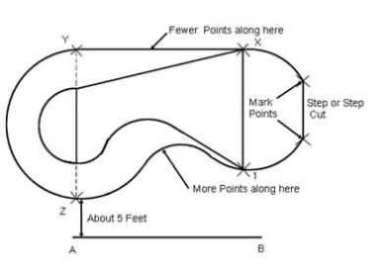Return

Measuring Bottom Dimensions

To help establish bottom dimensions, make sure to mark points where the break begins and ends. When measuring the back and side slopes use a point to measure from. Note which point was used and measure straight out from that point. Take depth measurements at the back of the hopper where the back wall starts and the sides of the hopper where the side walls start. Measure the depth of the shallow end and note if there is a cove or if the shallow end is not flat.

Return

Measuring Steps

• Always indicate what the corner type is on the back of the step (90 degree, radius, diagonal).
• Risers should equal shallow end wall depth.
• Measurements must be very precise.
• Always drain pool and cut out old liner to measure steps.
• If you are going to cover the step with foam we must know the thickness of the foam.
• If there is a setback indicate the type and size.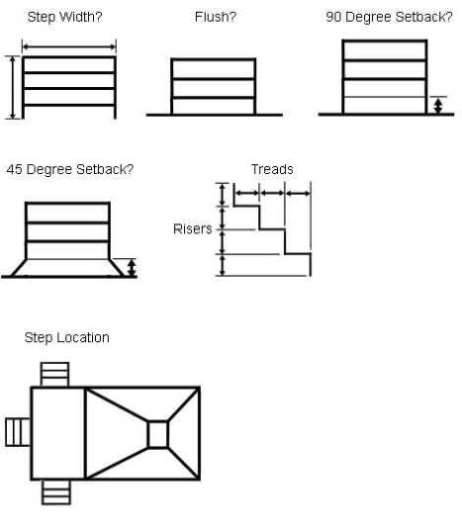Return

Squaring Corners

When measuring a pool it is helpful to "square up" radius or grecian (diagonal) corners. This becomes crucial when measuring True-L or Lazy-L pools, or when taking diagonal measurements. By squaring up the corners, you accomplish four things.

1. You have a common point of reference for length, width, and diagonal measurements.
2. By examining the completed pool measurements you can quickly determine if the pool is actually square.
3. If the pool is square you can accurately determine the corner size.
4. It is much easier for us to reconstruct the measurements into a precise pool shape.

To square up a corner you need to extend the straight wall past the corner by snapping a chalk line. You need to do the same with the other straight wall that makes up the corner. Make sure to line up the string for the chalk line with the bead track. Do not line it up with the edge of the coping since the bead track is not right on the edge. Where your two chalk lines intersect should be used as your point of reference for measuring.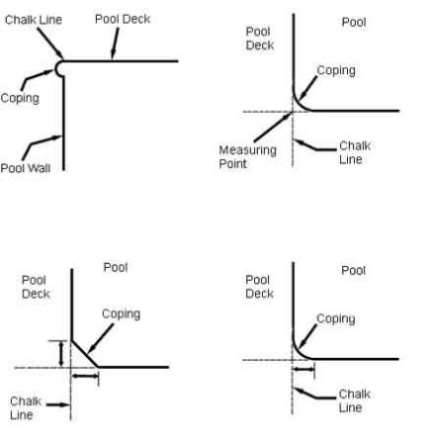Return

Measuring Roman End Pools

Roman End pools require more measurements than an ordinary rectangular pool. This involves measurements for both radius ends, all corner sections straight walls and hopper dimensions. See illustration 1 for perimeter measurements that should be taken.

On most Roman End pools with oval shaped hoppers additional measurements are needed. If there is a straight section on either side of the hopper we need to know what the straight section measures.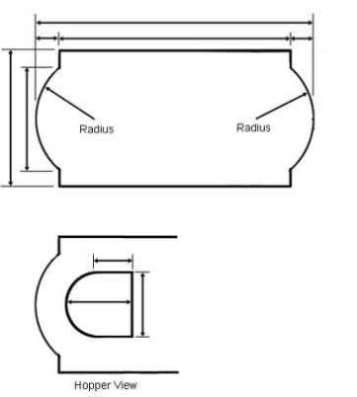Return

Posted on 05/18/2017 by Randy Johnson 5200

Blog archives